# 9个最常见IE的Bug及其fix

crazyinsomnia 发布于 2010/01/24 22:15

Internet Explorer – Web程序员的毒药。在IE上开发时间中有超过60%的时间是花在和IE的bug进行搏斗，让你的开发生产率严重下降。下面是一个教程，告诉你9个IE上最常见的BUG以及如何解决它们。

#### 1. 居中布局

 `#container{`
 `    ``border``: ``solid` `1px` `#000``;`
 `    ``background``: ``#777``;`
 `    ``width``: ``400px``;`
 `    ``height``: ``160px``;`
 `    ``margin``: ``30px` `0` `0` `30px``;`
 `}`
 `#element{`
 `    ``background``: ``#95CFEF``;`
 `    ``border``: ``solid` `1px` `#36F``;`
 `    ``width``: ``300px``;`
 `    ``height``: ``100px``;`
 `    ``margin``: ``30px` `auto``;`
 `}`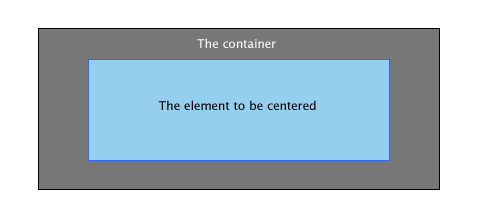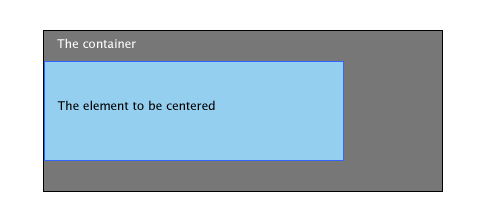`#container{`
 `    ``border``: ``solid` `1px` `#000``;`
 `    ``background``: ``#777``;`
 `    ``width``: ``400px``;`
 `    ``height``: ``160px``;`
 `    ``margin``: ``30px` `0` `0` `30px``;`
 `    ``text-align``: ``center``;`
 `}`
 `#element{`
 `    ``background``: ``#95CFEF``;`
 `    ``border``: ``solid` `1px` `#36F``;`
 `    ``width``: ``300px``;`
 `    ``height``: ``100px``;`
 `    ``margin``: ``30px` `0``;`
 `    ``text-align``: ``left``;`
 `}`

#### 2. 楼梯式的效果

 `<``ul``>`
 `    ``<``li``><``a` `href``=``"#"``>`
 `    ``<``li``><``a` `href``=``"#"``>`
 `    ``<``li``><``a` `href``=``"#"``>`
 ``

 `ul {`
 `    ``list-style``: ``none``;`
 `}`
 `ul li a {`
 `    ``display``: ``block``;`
 `    ``width``: ``130px``;`
 `    ``height``: ``30px``;`
 `    ``text-align``: ``center``;`
 `    ``color``: ``#fff``;`
 `    ``float``: ``left``;`
 `    ``background``: ``#95CFEF``;`
 `    ``border``: ``solid` `1px` `#36F``;`
 `    ``margin``: ``30px` `5px``;`
 `}`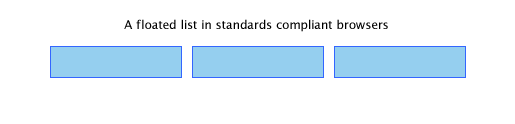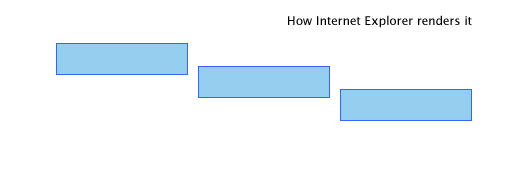`ul li {`
 `    ``float``: ``left``;`
 `}`

 `ul li {`
 `    ``display``: ``inline`
 `}`

#### 3. float元件的两倍空白

 `#element{`
 `    ``background``: ``#95CFEF``;`
 `    ``width``: ``300px``;`
 `    ``height``: ``100px``;`
 `    ``float``: ``left``;`
 `    ``margin``: ``30px` `0` `0` `30px``;`
 `    ``border``: ``solid` `1px` `#36F``;`
 `}`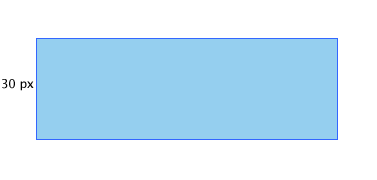IE的结果是：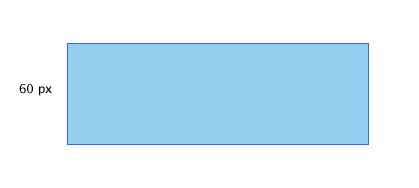`#element{`
 `    ``background``: ``#95CFEF``;`
 `    ``width``: ``300px``;`
 `    ``height``: ``100px``;`
 `    ``float``: ``left``;`
 `    ``margin``: ``30px` `0` `0` `30px``;`
 `    ``border``: ``solid` `1px` `#36F``;`
 `    ``display``: ``inline``;`
 `}`

#### 4. 无法设置微型高度

 `#element{`
 `    ``background``: ``#95CFEF``;`
 `    ``border``: ``solid` `1px` `#36F``;`
 `    ``width``: ``300px``;`
 `    ``height``: ``2px``;`
 `    ``margin``: ``30px` `0``;`
 `}`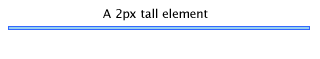IE的结果：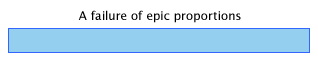`#element{`
 `    ``background``: ``#95CFEF``;`
 `    ``border``: ``solid` `1px` `#36F``;`
 `    ``width``: ``300px``;`
 `    ``height``: ``2px``;`
 `    ``margin``: ``30px` `0``;`
 `    ``font-size``: ``0``;`
 `}`

 `#element{`
 `    ``background``: ``#95CFEF``;`
 `    ``border``: ``solid` `1px` `#36F``;`
 `    ``width``: ``300px``;`
 `    ``height``: ``2px``;`
 `    ``margin``: ``30px` `0``;`
 `    ``overflow``: ``hidden`
 `}`

#### 5. 跨出边界

 `<``div` `id``=``"element"``><``div` `id``=``"anotherelement"``>`

 `#element{`
 `    ``background``: ``#95CFEF``;`
 `    ``border``: ``solid` `1px` `#36F``;`
 `    ``width``: ``300px``;`
 `    ``height``: ``150px``;`
 `    ``margin``: ``30px` `0``;`
 `    ``overflow``: ``auto``;`
 `}`
 `#anotherelement{`
 `    ``background``: ``#555``;`
 `    ``width``: ``150px``;`
 `    ``height``: ``175px``;`
 `    ``position``: ``relative``;`
 `    ``margin``: ``30px``;`
 `}`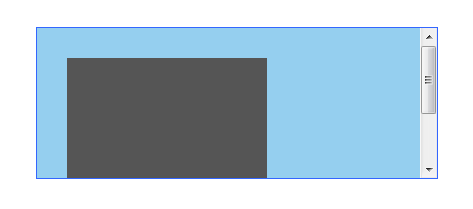IE的结果：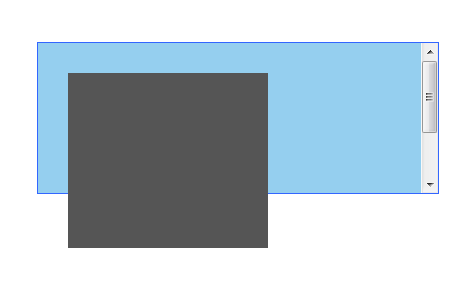`#element{`
 `    ``background``: ``#95CFEF``;`
 `    ``border``: ``solid` `1px` `#36F``;`
 `    ``width``: ``300px``;`
 `    ``height``: ``150px``;`
 `    ``margin``: ``30px` `0``;`
 `    ``overflow``: ``auto``;`
 `    ``position``: ``relative``;`
 `}`

#### 6. Fixing the Broken Box Model

Internet Explorer曲解了“盒子模子”可能是最不可原谅的事情了。IE 6 这个半标准的浏览器回避了这个事情，但这个问题还是会因为IE运行在“怪异模式”下出现。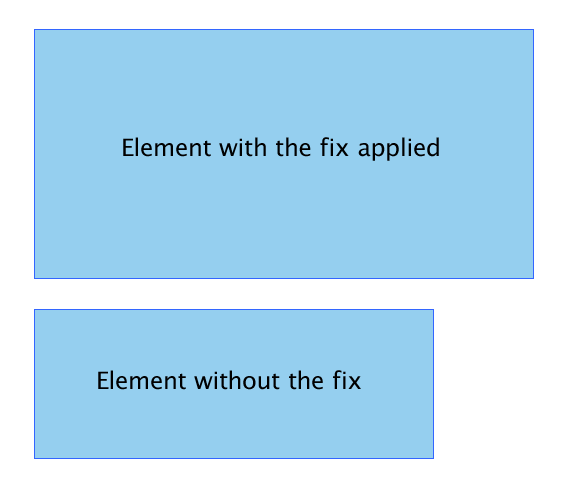`#element{`
 `    ``width``: ``400px``;`
 `        ``height``: ``150px``;`
 `    ``padding``: ``50px``;`
 `}`

 `#element {`
 `    ``width``: ``400px``;`
 `    ``height``: ``150px``;`
 `    ``height``: ``250px``;`
 `    ``width``: ``500px`
 `}`

#### 7. 设置min-height和min-width

IE忽略了min-height。

 `#element {`
 `  ``min-height``:``150px``;`
 `  ``height``:``auto` `!important``;`
 `  ``height``:``150px``;`
 `}`

 `#element {`
 `    ``min-height``: ``150px``;`
 `    ``height``: ``150px``;`
 `}`
 `html>body #element {`
 `    ``height``: ``auto``;`
 `}`

#### 8. Float 布局错误行为 Misbehaving

 `<``div` `id``=``"container"``>`
 `    ``<``div` `id``=``"element"``>http://net.tutsplus.com/`
 `    ``<``div` `id``=``"anotherelement"``>`
 ``

 `#element, #anotherelement{`
 `    ``background``: ``#95CFEF``;`
 `    ``border``: ``solid` `1px` `#36F``;`
 `    ``width``: ``100px``;`
 `    ``height``: ``150px``;`
 `    ``margin``: ``30px``;`
 `    ``padding``: ``10px``;`
 `    ``float``: ``left``;`
 `}`
 `#container{`
 `    ``background``: ``#C2DFEF``;`
 `    ``border``: ``solid` `1px` `#36F``;`
 `    ``width``: ``365px``;`
 `    ``margin``: ``30px``;`
 `    ``padding``: ``5px``;`
 `    ``overflow``: ``auto``;`
 `}`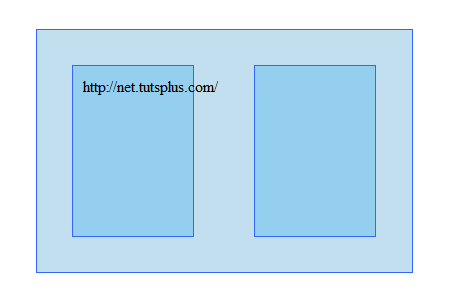IE的结果：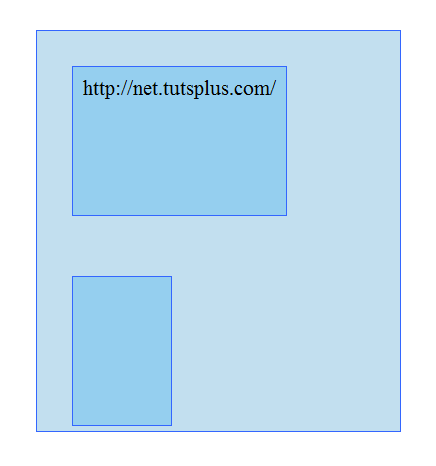`#element{`
 `    ``background``: ``#C2DFEF``;`
 `    ``border``: ``solid` `1px` `#36F``;`
 `    ``width``: ``365px``;`
 `    ``margin``: ``30px``;`
 `    ``padding``: ``5px``;`
 `    ``overflow``: ``hidden``;`
 `}`

#### 9. 在list项目门的空行

 `<``ul``>`
 ` ``<``li``><``a` `href``=``"#"``>Link 1`
 ` ``<``li``><``a` `href``=``"#"``>Link 2`
 ` ``<``li``><``a` `href``=``"#"``>Link 3`
 ``

 `ul {`
 `    ``margin``:``0``;`
 `    ``padding``:``0``;`
 `    ``list-style``:``none``;`
 `}`
 `li a {`
 `    ``background``: ``#95CFEF``;`
 `    ``display``: ``block``;`
 `}`IE的结果：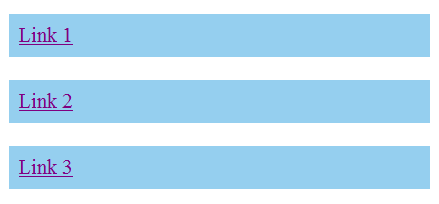Fortunately, there are a plethora of fixes you could try.

 `li a {`
 `    ``background``: ``#95CFEF``;`
 `    ``display``: ``block``;`
 `    ``height``: ``200px``;`
 `}`

 `li a {`
 `    ``background``: ``#95CFEF``;`
 `    ``float``: ``left``;`
 `    ``clear``: ``left``;`
 `}`

li 加上display: inline

 `li {`
 `    ``display``: ``inline``;`

`}`

#### 结论